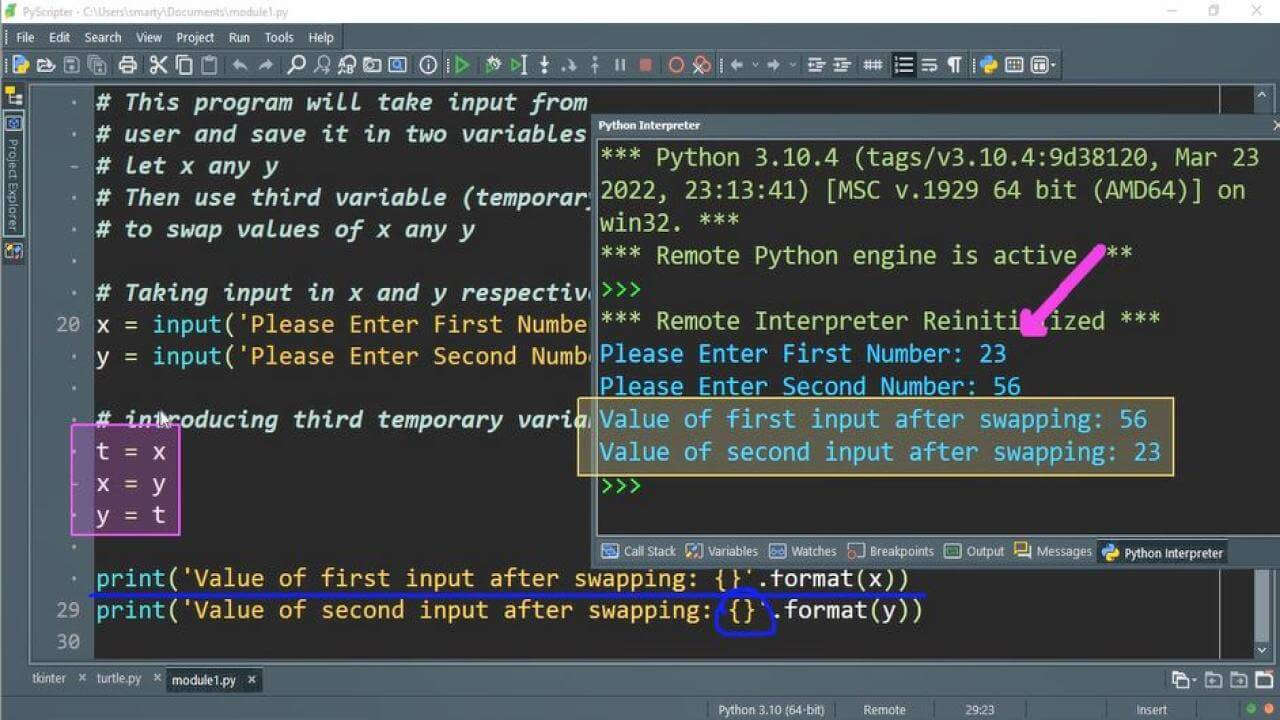# Write a Program to Swap Two Numbers In Python

## Source Code:

In python there are multiple way to swap values of variable. We can swamp values using third variable, using arithmetic operation and by using assignment statement. We will discuss here these all three methods.

### Swap using temp variable:

# This program will take input from
# user and save it in two variables
# let x any y
# Then use third variable (temporary variable)
# to swap values of x any y

# Taking input in x and y respectively
x = input('Please Enter First Number: ')
y = input('Please Enter Second Number: ')

# introducing third temporary variable t
t = x
x = y
y = t

print('Value of first input after swapping: {}'.format(x))
print('Value of second input after swapping: {}'.format(y))

### Swap using arithmetic operation:

We can also swap value of two variables without using third/temp variable. In this way we have to perform some arithmetic operations on given variables.

For performing arithmetic operations on input we must have to use cast operator, beacuse in python input is of string type. We cannot perform arithmetic operations without using cast operator in python.

# This program will interchange
# values of two variables
# let a any b
# Without using third variable

# Taking input in variables a and b
a = input('Input First Number: ')
b = input('Input Second Number: ')

# performing arithmetic operation
a = int(a) + int(b)
b = int(a) - int(b)
a = int(a) - int(b)

print('First value after swapping: {}'.format(a))
print('Second value after swapping: {}'.format(b))

### Swap using assignment statement:

In python there is also any easy and quick way for swapping values of variables. We just use assignment statement provided variables as Lvalue and Rvalue separated by comma.

# This program will swap
# two variables values
# let say value of a any b
# using assignment statement

# taking a,b input using input function
a = input('First Input: ')
b = input('Second Input: ')

# pyhton swap using assignment operator
a, b = b, a

print('Value of a after swap: {}'.format(a))
print('Value of b after swap: {}'.format(b))

## Output:

Output of program using third variable will be as: